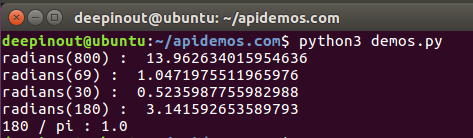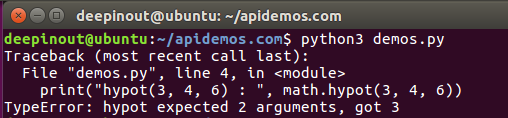# Python Math hypot() 函数

## Python Math hypot() 函数

Python Math hypot() 返回欧几里德范数 sqrt(xx + yy)。

## Python Math hypot() 语法

import math

math.hypot(x, y)

• x : 一个数值。
• y : 一个数值。

## Python Math hypot() 示例1

#!/usr/bin/python3
import math

print ("hypot(6, 4) : ",  math.hypot(6, 4))
print ("hypot(-6, 6) : ",  math.hypot(-6, 6))
print ("hypot(0, 2) : ",  math.hypot(0, 2))## Python Math hypot() 示例2

hypot()函数中的错误。

#!/usr/bin/python3
import math

print("hypot(3, 4, 6) : ", math.hypot(3, 4, 6))• 回顶